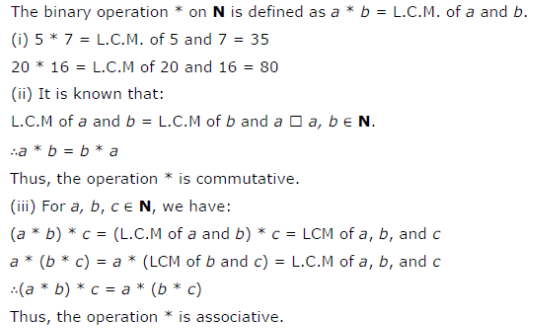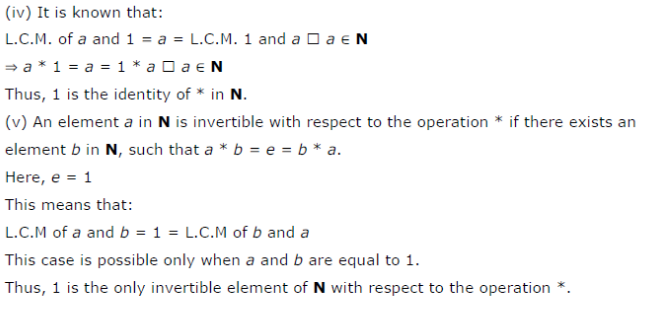# Let * be the binary operation on N given by a * b = L.C.M. of a and b Find:

Let * be the binary operation on N given by a * b = L.C.M. of a and b Find:
(i) 5 * 7, 10 * 16
(ii) Is * commutative?
(iii) Is * associative?
(iv) Find the identity of * in N.
(v) Which elements of N are invertible for the operation *?